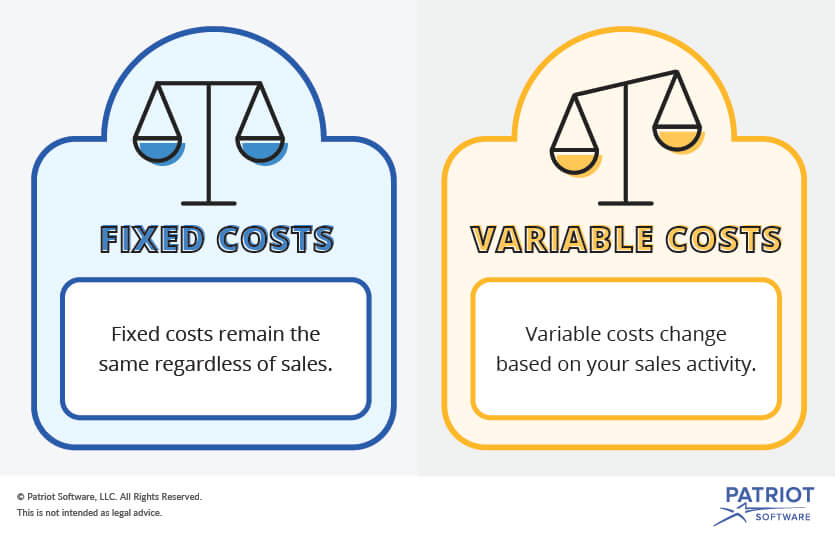Do You Know the Difference Between Fixed vs. Variable Costs?

# Fixed vs. Variable Costs

Business expenses are categorized as either fixed or variable costs. You should identify your company’s fixed and variable expenses for accurate books. Learn the difference between fixed vs. variable costs to help with budgeting, pricing, and decision making.

## Fixed vs. variable costs

Fixed costs are expenses that remain the same, regardless of how many sales you make. Fixed costs are expenses you must pay to run your business. You will always have fixed costs, even if you don’t sell much. Generally, your fixed expenses will stay the same from month to month.

On the other hand, variable costs fluctuate based on your sales activity. If sales are high, your variable costs increase. And if sales are low, your variable costs decrease. Variable expenses change from month to month.### Fixed and variable costs examples list

Understanding which of your expenses are fixed and which are variable is important. To help, take a look at these fixed and variable costs examples.

Here are a few examples of fixed costs you might have in your business:

• Rent
• Insurance
• Loan payments

For example, if you rent business space, you have a regularly recurring payment each month, regardless of how many sales you made. The amount is the same unless your lease agreement changes.

Here are examples of variable costs you could have in your business:

• Direct materials
• Direct labor
• Commissions

Let’s say your employee earns commissions. The more they sell, the higher the amount you owe them. Their wages are a variable cost because they depend on sales.

## Using fixed and variable costs in your pricing structure

### How to find your total costs

To find your business’s total costs, you need to know both fixed cost and variable cost. Finding fixed costs is straightforward because they are the same each month. But to find your total variable costs, you need to use the variable cost formula.

#### Variable cost formula

You need to know how many products you sold and the variable cost of each product to use the variable cost formula.

Total Variable Costs = Products Sold X Variable Cost Per Unit

Let’s say you sold 5,000 cell phone cases. It costs you \$5 to make each case. Your total variable costs are \$25,000.

#### Finding total costs

Total Costs = Fixed Costs + Variable Costs

However, you should also know how many variable and fixed costs you spend on each unit. This can help you set a fair price that results in a profit for you. Here is the total cost per unit formula.

Total Cost Per Unit = (Fixed Costs + Variable Costs) / Total Units Produced

For example, you have \$5,000 in fixed costs and \$3,000 in variable costs. You produced 4,000

Total Cost Per Unit = (\$5,000 + \$3,000) / 4,000

Total Cost Per Unit = \$2

You spend \$2 making each unit. You can use this information to set a price for each product.

## Mixed costs

Not all expenses are just fixed or variable. Some expenses might have aspects of both fixed and variable costs. This kind of expense is known as a mixed, semi-variable, or semi-fixed cost.

Mixed costs are fixed up until a certain point. Then, they become variable.

Examples of mixed costs include salaried (fixed) workers who also receive commissions (variable) or work overtime (variable) and car expenses like a monthly lease (fixed) and gas (variable).

Let’s say an employee needs to work overtime as a result of increased sales. Because the overtime wages are due to a rise in sales, they are variable. But, the employee’s regular wages are fixed costs. This would make the employee’s total pay for that pay period (overtime and regular) a mixed cost.

Looking for an easy way to track your business’s fixed and variable costs? Patriot’s online accounting software uses a simple system that is made for the non-accountant to simplify expense tracking. Try it for free today!